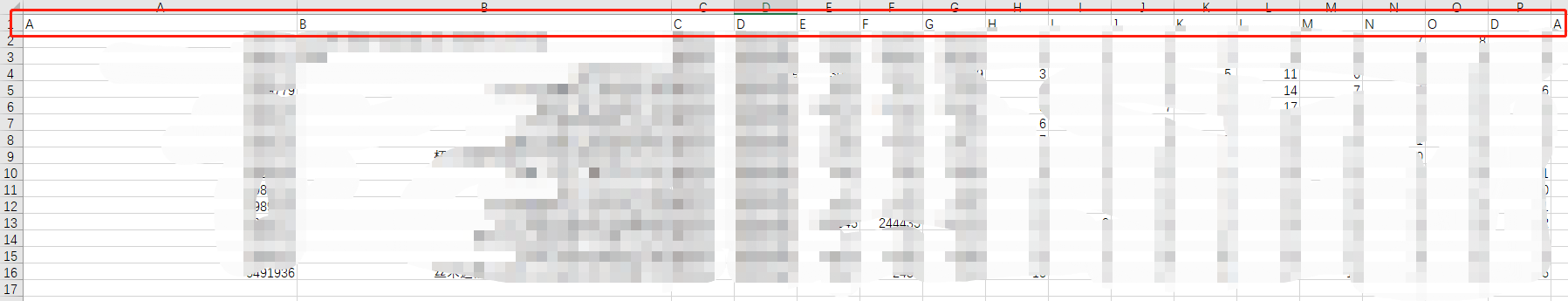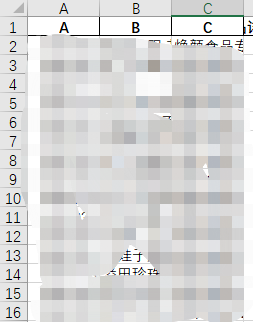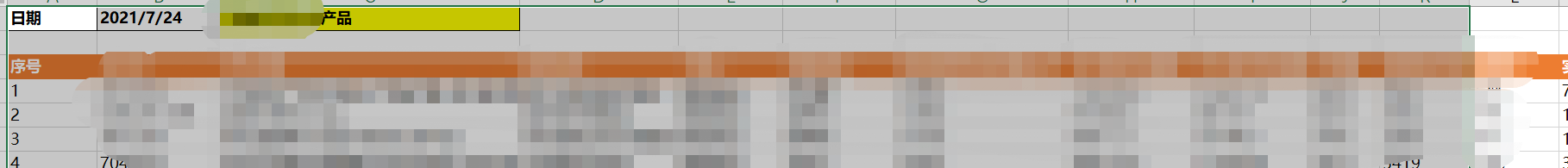# 使用pandas处理excel，并使用Openpyxl修改单元格格式

## 需求## 处理基本流程

1. 利用pandas读取表格数据
2. 根据某几行，生成新的指标，将新的指标添加到表格中
3. 筛选出需要的列
4. 添加标题栏，设置部分单元格格式

## 查询资料过程

**工具：**最初使用jupyter,因为方便查看中间过程，方便调试，最后使用pycharm一键处理。

#### 1.读取数据，

df = pd.read_excel(path_data,sheet_name=sheet_original)


#### 2.指标的计算：计算较为简单，新指标由某几列的四则运算生成，放代码：

df['A'] = (df['B'] / df['C'])
df['D'] =((df['A'] - df['C']) / df['A'])


df['A'] = df['A'].round(decimals=2)
df['B'] = df['B'].round(decimals=2)


df['A'] = df['A'].map(lambda x : format(x,'.2%'))


#### 3.筛选需要的列：新的df列索引的顺序会按照代码中顺序生成

df1 = df[['A','B','C']]


ascending是决定升序（True）降序(False)。

#将原数据排序
#拷贝一份新的数据


#### 4.如何保存dataframe到新的sheet

#保存操作 会覆盖原数据,去除索引
df2.to_excel('测试.xlsx',sheet_name = 'topn'，index = False)

#保存操作，仍然是覆盖，只不过是原页又保存了一遍
with pd.ExcelWriter('2.xlsx') as writer:
df.to_excel(writer, sheet_name='data')
df.to_excel(writer, sheet_name='data2')

#保存操作，不覆盖（真）
with pd.ExcelWriter(path_data, engine='openpyxl', mode='a') as writer:
df2.to_excel(writer,sheet_name=sheet_name1,index=False)
df3.to_excel(writer, sheet_name=sheet_name2, index=False)


## 样式调整，设置字体、颜色、标题栏1. 最上方有日期，说明 等标题栏，并且背景填充，加粗。
2. 序号列（这个最初保存的时候就有，我给它取消了），但是原本保存的序号是从0开始，这里需要改为从1开始。
3. 列索引一行背景填充
4. 格式的设置：字体微软雅黑，左对齐。

#### 1.插入标题和日期

df2.to_excel(writer,sheet_name=sheet_1,index=True,header=True, startrow=2)

ws.cell(row=1, column=1, value='日期')
ws.cell(row=1, column=2, value=today)
ws.cell(row=1, column=3, value=sheet_1+'产品')
ws.cell(row = 3,column=1,value='序号')


#### 2.排序后索引会乱，所以这里需要索引重新开始，并从1开始

#索引重新排序，并从1开始
df2.reset_index(drop=True, inplace=True)
df2.index = np.arange(1,len(df2)+1)


#### 3和4放在一起，都是使用openpyxl设置格式

https://zhuanlan.zhihu.com/p/261962080


def set_cells(cells,type,color=000000):
aligncenter=Alignment(horizontal='center',vertical='center')  #居中

# border=Border(left=sidestyle,right=sidestyle,top=sidestyple,bottom=sidestyle)
for i in cells:
for j in i:
if(type=='percent'):
j.number_format='0.00%'
elif(type=='bold'):
j.style='Pandas'
elif(type=='center'):
j.alignment=aligncenter
elif(type=='color'):
j.font = Font(color=color)  #solid为样式
elif(type=='fill'):
j.fill = PatternFill(patternType="solid", start_color=color)  # 纯色填充


## 最后附上全文代码，如果有错误请大家指教，会及时改正的，代码写的比较烂，大家不要介意。由于实习原因，里边的列索引我都把改成了ABCD,数量可能和图文的图片对应不上，大家只要改成自己的数据就可以了，环境的安装也比较简单，就不赘述了。

# 导入必要的包
import pandas as pd
from openpyxl.styles import Font, Border, Side, PatternFill, Alignment
import datetime
import numpy as np

def set_cells(cells, type, color=000000):
aligncenter = Alignment(horizontal='left', vertical='center')  # 居中

# border=Border(left=sidestyle,right=sidestyle,top=sidestyple,bottom=sidestyle)
for i in cells:
for j in i:
j.font=Font(name = u'微软雅黑')
if (type == 'percent'):
j.number_format = '0%'
elif (type == 'bold'):
j.style = 'Pandas'
elif (type == 'left'):
j.alignment = aligncenter
elif (type == 'color'):
j.font = Font(color=color)  # solid为样式
elif (type == 'fill'):
j.fill = PatternFill(patternType="solid", start_color=color)  # 纯色填充

def main():

# 数据处理生成
df['A'] = df['B'] / df['C']
df['D'] = df['E'] / df['F）']
df['X'] = df['A'] / df['B']
df['Y'] = (df['A'] - df['B']) / df['A']

# 保留两位小数
df['A'] = df['A'].round(decimals=2)
df['A'] = df['B'].round(decimals=2)

# 筛选需要的表格，excel的列会按照这个顺序生成

df1 = df[['A', 'B', 'C', ...]]
# 重新对序号排序,索引从1开始
df2.reset_index(drop=True, inplace=True)
df2.index = np.arange(1,len(df2)+1)
df3.reset_index(drop=True, inplace=True)

# 保存操作，不覆盖
with pd.ExcelWriter(path_data, engine='openpyxl', mode='a') as writer:
ws = writer.sheets[sheet_1]

# 插入标题：日期、说明
ws.cell(row=1, column=1, value='日期')
ws.cell(row=1, column=2, value=today)
ws.cell(row=1, column=3, value=sheet_2 + '产品')
ws.cell(row=3, column=1, value='序号')

# 设置字体与单元格格式
set_cells(ws['A1:C1'], 'bold')
set_cells(ws['A1:M53'], 'left')
set_cells(ws['A3:O3'], 'fill', 'FF8C00')
set_cells(ws['C1:C1'], 'fill', 'FFFF00')

# 设置为百分数
set_cells(ws['L4:L53'], 'percent')
set_cells(ws['O4:O53'], 'percent')

ws = writer.sheets[sheet_2]
ws.cell(row=1, column=1, value='日期')
ws.cell(row=1, column=2, value=today)
ws.cell(row=1, column=3, value=sheet_2 + '产品')
ws.cell(row=3, column=1, value='序号')

# 设置字体与单元格格式
set_cells(ws['A1:C1'], 'bold')
set_cells(ws['A1:M53'], 'left')
set_cells(ws['A3:O3'], 'fill', 'FF8C00')
set_cells(ws['C1:C1'], 'fill', 'FFFF00')

# 设置为百分数
set_cells(ws['L4:L53'], 'percent')
set_cells(ws['O4:O53'], 'percent')

if __name__ == "__main__":
# 数据源路径  页面名称
path_data = r'xxx.xlsx'
sheet_original = r'sheet_name'
today = str(datetime.date.today())
# 保存页面名称
sheet_1 = '1TOPn'
sheet_2 = '2TOPn'

main()

11-27551

07-131674
09-30117
12-291249
04-023483
02-243186
03-111万+
03-126705
12-311439
01-02285
03-02506
03-2886
12-22321
12-061352
07-042029
11-241015
04-062021

### “相关推荐”对你有帮助么？

•非常没帮助
•没帮助
•一般
•有帮助
•非常有帮助weixin_42523992

¥2 ¥4 ¥6 ¥10 ¥20余额支付 (余额：-- )扫码支付获取中扫码支付点击重新获取扫码支付1.余额是钱包充值的虚拟货币，按照1:1的比例进行支付金额的抵扣。
2.余额无法直接购买下载，可以购买VIP、C币套餐、付费专栏及课程。余额充值